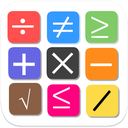# Maths King - Learn all maths in one App

نسخهٔ ۱۳.۲۳
+۱۰
نصب فعال
آموزش
دسته‌بندی
۵ مگابایت
حجم# Maths King - Learn all maths in one App

LemdaSolutions
نسخهٔ ۱۳.۲۳
+۱۰
نصب فعال
آموزش
دسته‌بندی
۵ مگابایت
حجم

## معرفی برنامه

جزئیات بیشتر

Maths King app help you to Improve your mathematics skills and you can sharp you mind in all kinds of mathematics problems.
This mathematics app has all the maths functionality to grab and learn mathematics in easy and fun way.
Maths king is a fully functional application for every one who want to improve their math skills with fun and ease.

Maths king contains Math Tricks, Math Formulas, Math Definitions, Math Games, Math quizzes, Math puzzles, maths, Math Symbols

Features
------------
Number Learning feature, Learn addition subtraction multiplication division and percentage calculation in a fun way.
Also learn and practice solving the equation.
There are Roman number learning tutorial provided.
having many maths tables auto generation feature provided.

Learn about converting number into words.
There many game inbuilt in this Application like :
SUDOKU game, puzzles game, Alphabets Quiz, Months Quiz, Shapes Learning

There many more Quizes available. You can practice in those quizs
Times Quiz, Week Days Quiz, Counter Quiz, Range Quiz, Math units, Chocolate Quiz, Calculation Quiz, Binary Hex Decimal number, Paint, Odd Even Quiz, Decimal Quiz
Word to Number Quiz, Fill in the Blanks, Addition table, Area formulas, Circle details
information,

Maths King also covers all the formula required in school as well as college.

This app covers all the fundamental maths concepts which you can learn practice and improve by your own.
Algebra Basics, Algebra Exponents, Number Notation , Length and Distance Conversion Table

Further Maths king has a different conversion tables.
In addition there are many maths shortcut tricks are there for faster calculation and rapid solution.

Even you can learn different numbers system using this App i.e Binary Numbers, Hexadecimal Numbers, Octal Numbers, Roman Numbers etc.

There are interesting different levels of mathematical operations.
Equation solving and Remainder questions are very interesting for those who interested in Maths Puzzle.
Also a very exciting feature to learn ROMAN NUMBERS, as well as wordings for multiple zero numbers words.
Maths King Further provide a Puzzle game which can improve maths of all type users . which provide a mathematical challenge in each question.
And yes s Arrange Number game is there which can Improve Maths skills.

It contains almost all the mathematics which you required to improve your mathematics.
It has mathematics Units, Mathematics Games, Mathematics Puzzles, Maths Formulas and many more.

نظرات کاربران - ۰ رأی
۰ از ۵
۵
۴
۳
۲
۱